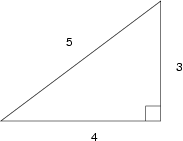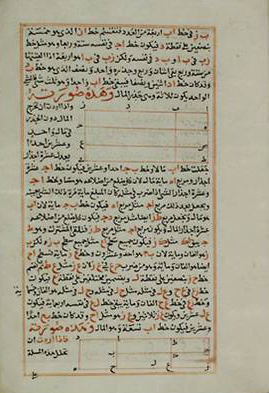# A Trillion Triangles

September 22, 2009 -- Mathematicians from North America, Europe, Australia, and South America have resolved the first one trillion cases of an ancient mathematics problem. The advance was made possible by a clever technique for multiplying large numbers. The numbers involved are so enormous that if their digits were written out by hand they would stretch to the moon and back. The biggest challenge was that these numbers could not even fit into the main memory of the available computers, so the researchers had to make extensive use of the computers' hard drives.

According to Brian Conrey, Director of the American Institute of Mathematics, "Old problems like this may seem obscure, but they generate a lot of interesting and useful research as people develop new ways to attack them."

 Press release in MSWord or plain text. Versión en español

 Computer details: how to multiply large numbers

The problem, which was first posed more than a thousand years ago, concerns the areas of right-angled triangles. The surprisingly difficult problem is to determine which whole numbers can be the area of a right-angled triangle whose sides are whole numbers or fractions. The area of such a triangle is called a "congruent number." For example, the 3-4-5 right triangle which students see in geometry has area 1/2 × 3 × 4 = 6, so 6 is a congruent number. The smallest congruent number is 5, which is the area of the right triangle with sides 3/2, 20/3, and 41/6.The 3-4-5 triangle has area 6.

The first few congruent numbers are 5, 6, 7, 13, 14, 15, 20, and 21. Many congruent numbers were known prior to the new calculation. For example, every number in the sequence 5, 13, 21, 29, 37, ..., is a congruent number. But other similar looking sequences, like 3, 11, 19, 27, 35, ...., are more mysterious and each number has to be checked individually.

The calculation found 3,148,379,694 of these more mysterious congruent numbers up to a trillion.

 Math details: triangles and elliptic curves

## Consequences, and future plans

Team member Bill Hart noted, "The difficult part was developing a fast general library of computer code for doing these kinds of calculations. Once we had that, it didn't take long to write the specialized program needed for this particular computation." The software used for the calculation is freely available, and anyone with a larger computer can use it to break the team's record or do other similar calculations.

In addition to the practical advances required for this result, the answer also has theoretical implications. According to mathematician Michael Rubinstein from the University of Waterloo, "A few years ago we combined ideas from number theory and physics to predict how congruent numbers behave statistically. I was very pleased to see that our prediction was quite accurate." It was Rubinstein who challenged the team to attempt this calculation. Rubinstein's method predicts around 800 billion more congruent numbers up to a quadrillion, a prediction that could be checked if computers with a sufficiently large hard drive were available.

## History of the problem

The congruent number problem was first stated by the Persian mathematician al-Karaji (c.953 - c.1029). His version did not involve triangles, but instead was stated in terms of the square numbers, the numbers that are squares of integers: 1, 4, 9, 16, 25, 36, 49, ..., or squares of rational numbers: 25/9, 49/100, 144/25, etc. He asked: for which whole numbers n does there exist a square a2 so that a2-n and a2+n are also squares? When this happens, n is called a congruent number. A major influence on al-Karaji was the Arabic translations of the works of the Greek mathematician Diophantus (c.210 - c.290) who posed similar problems.Al-Fakhri fi'l-jabr wa'l-muqabala, by al-Karaji.
A small amount of progress was made in the next thousand years. In 1225, Fibonacci (of "Fibonacci numbers" fame) showed that 5 and 7 were congruent numbers, and he stated, but did not prove, that 1 is not a congruent number. That proof was supplied by Fermat (of "Fermat's last theorem" fame) in 1659. By 1915 the congruent numbers less than 100 had been determined, and in 1952 Kurt Heegner introduced deep mathematical techniques into the subject and proved that all the prime numbers in the sequence 5, 13, 21, 29,... are congruent. But by 1980 there were still cases smaller than 1000 that had not been resolved.

### Modern results

In 1982 Jerrold Tunnell of Rutgers University made significant progress by exploiting the connection (first used by Heegner) between congruent numbers and elliptic curves, mathematical objects for which there is a well-established theory. He found a simple formula for determining whether or not a number is a congruent number. This allowed the first several thousand cases to be resolved very quickly. One issue is that the complete validity of his formula (therefore also the new computational result) depends on the truth of a particular case of one of the outstanding problems in mathematics known as the Birch and Swinnerton-Dyer Conjecture. That conjecture is one of the seven Millennium Prize Problems posed by the Clay Math Institute with a prize of one million dollars.

 Math basics: congruences and "modulo"

## The computations

Results such as these are sometimes viewed with skepticism because of the complexity of carrying out such a large calculation and the potential for bugs in either the computer or the programming. The researchers took particular care to verify their results, doing the calculation twice, on different computers, using different algorithms, written by two independent groups. The team of Bill Hart (Warwick University, in England) and Gonzalo Tornaria (Universidad de la Republica, in Uruguay) used the computer Selmer at the University of Warwick. Selmer is funded by the Engineering and Physical Sciences Research Council in the UK. Most of their code was written during a workshop at the University of Washington in June 2008.

The team of Mark Watkins (University of Sydney, in Australia), David Harvey (Courant Institute, NYU, in New York) and Robert Bradshaw (University of Washington, in Seattle) used the computer Sage at the University of Washington. Sage is funded by the National Science Foundation in the US. The team's code was developed during a workshop at the Centro de Ciencias de Benasque Pedro Pascual in Benasque, Spain, in July 2009. Both workshops were supported by the American Institute of Mathematics through a Focused Research Group grant from the National Science Foundation.

 Details on the computers

 The technical paper describing the calculation

### Contact Information

 Media contact: Estelle Basor Deputy Director American Institute of Mathematics ebasor@aimath.org (650) 845-2071 Research contact: Bill Hart Research Fellow University of Warwick W.B.Hart@warwick.ac.uk